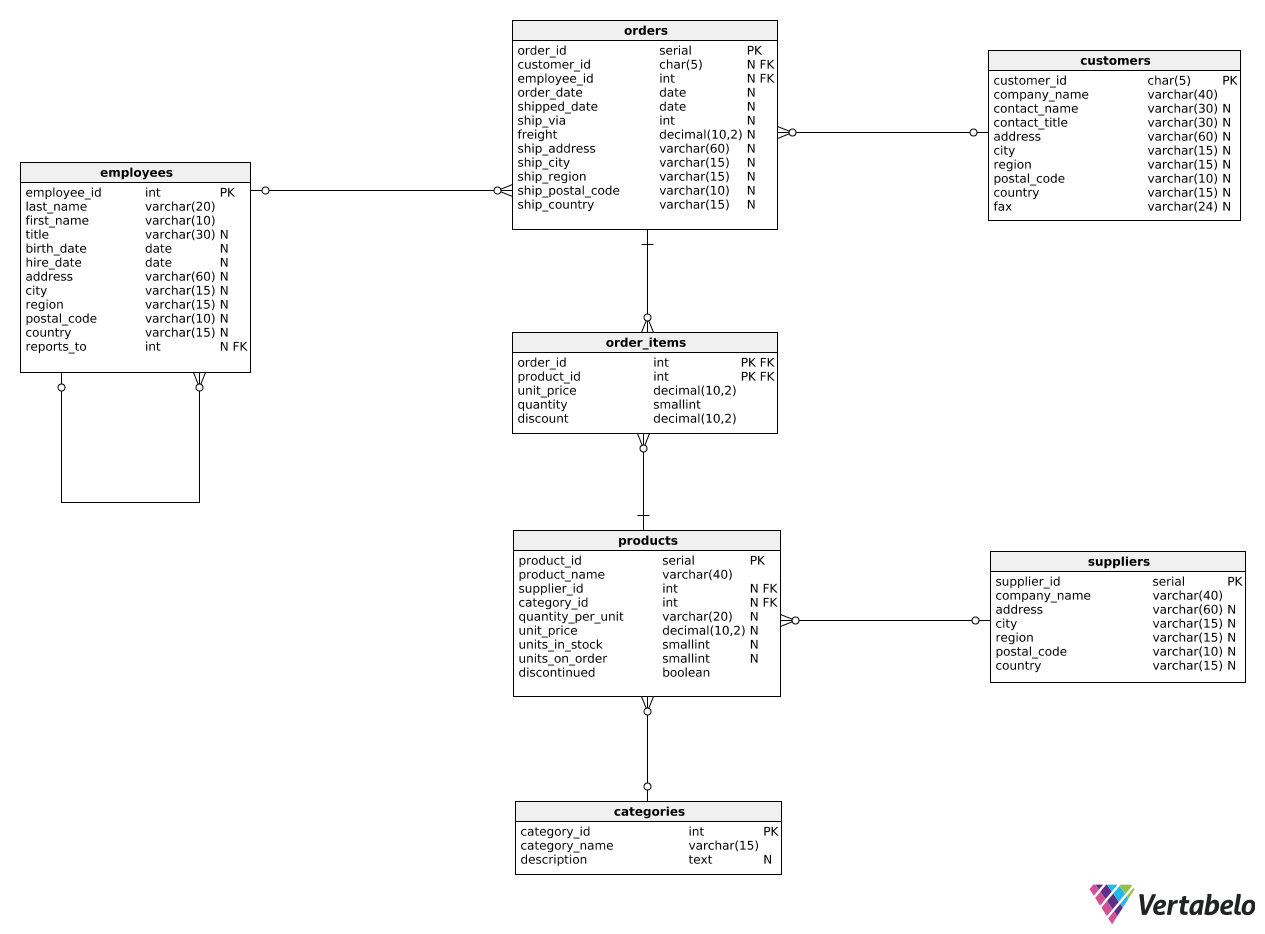Deals Of The Week - hours only!Up to 80% off on all courses and bundles.-Close
Introduction
Multiple metrics for a single object
Metrics for two groups
Ratios and percentages
8. Calculating ratios – step 3
Global vs. specific metrics
Summary

## Instruction

Good job! Even though we already computed the final ratio in step 2, we typically also want to round the ratio to a given number of decimal places. To that end, we can use the ROUND(value, decimal_places) function. Take a look:

SELECT
COUNT(CASE
WHEN shipped_date IS NOT NULL
THEN order_id
END) AS count_shipped,
COUNT(order_id) AS count_all,
ROUND(COUNT(CASE
WHEN shipped_date IS NOT NULL
THEN order_id
END) / COUNT(order_id)::decimal, 2) AS shipped_ratio
FROM orders;


We surrounded the last column with the ROUND(..., 2) function invocation. Because the second argument is 2, we'll get the ratio rounded to two decimal places.

## Exercise

Modify the template code. Round the ratio from the discounted_ratio column to three decimal places.

### Stuck? Here's a hint!

Wrap your division with a call to ROUND(..., 3).